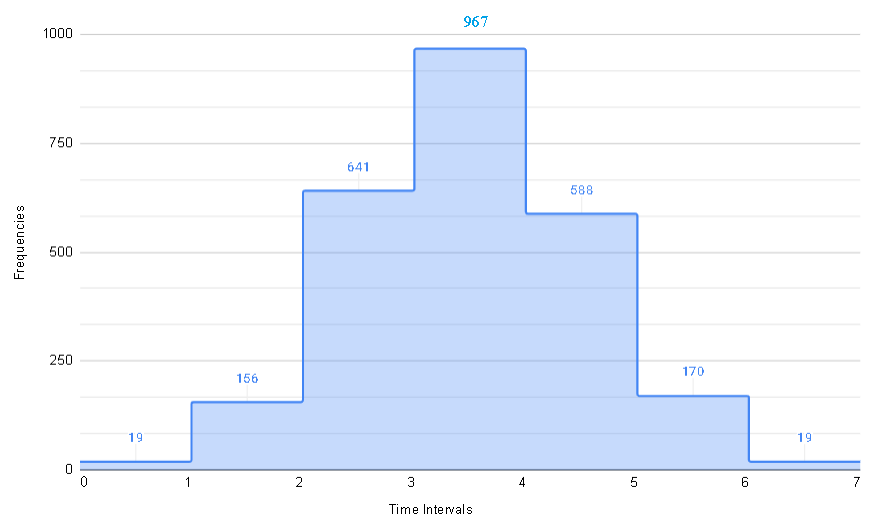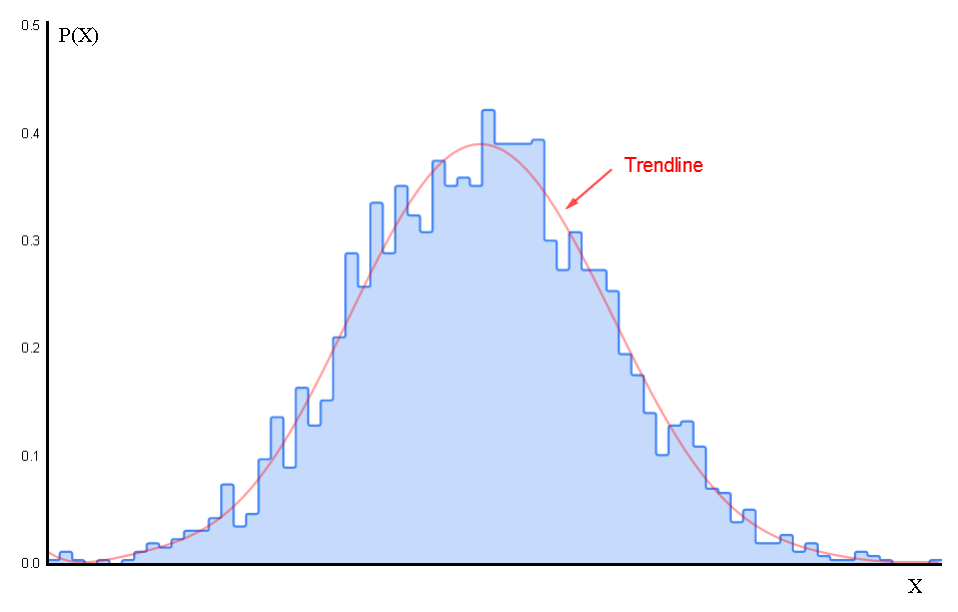# Probability Density for Continuous Variable

    

The definition of the probability density function is presented starting from a histogram , then a probability histogram . Examples with solutions related to probability density are also included.

## Histograms

We use 2560 data values generated using Google sheets to present the idea of moving from histogram of frequencies to a histogram of probabilities . The data represents the time (in hours) taken to finish a project and hence is assumed to be continuous.
The time here is considered as a continuous random variable.
The histogram obtained using google sheets is shown below. The classes on the horizontal axis have a width equal to 1 and the frequencies are on the vertical axis.
The sum of all frequencies is equal to the total number of data values which is equal to 2560.Figure 1

## From Histograms to Probability Distribution

### Class Width Equal to 1

We modify the above histogram into a probability histogram by dividing each frequency by the total number of data values which is 2560.
The probability histogram below tells us that if a data value is picked at random from the given data set, for example, the probability that this data value is greater than 3 and less than 4 is equal to 0.378 (rounded to 3 decimal places) which is the height of the rectangle whose width extends from x = 3 to x = 4.
The width of each rectangle makes the probability histogram is equal to 1 and therefore the area of each rectangle is equal to the height multiplied by the width which is equal to 1 and is the probability. So the probability histogram associates probability and area.
Note that the sum of all the probabilities in the histogram below is equal to 1 and therefore the total area of all rectangles is equal to 1.Figure 2

### Class Width Equal to 0.5

Because our data is continuous, we can use narrower width for the classes.
We now create another histogram of class width equal to 0.5. Again the heights give the probabilities and the sum of all probabilities is equal to the area of all the rectangles and is equal to 1.
We have again used Google sheets to add a "trendline" (shown in red) revealing the overall trend of the data based on the height of the rectangles in the probability histogram. We note that the area between the x-axis and the curve of this function (trendline) is closer to the total area under the rectangles.Figure 3

### Class Width Equal to 0.1

The class width is now equal to 0.1 and the trendline is even closer and the area between the x-axis and the curve of this function is even closer to the area under the rectangles.Figure 4

## Probability Density Function (PDF)

It is clear from the above probability histograms and the trendline, that if we have a large number of data values which is the case of a continuous random variable, as the class width becomes smaller, the trendline is a function that may be used to calculate probabilities using the area between the x-axis and the curve of this function.
This trendline function is called the probability density function.
If function $f_{X}(x)$ is the probability density function of a random variable $X$, then the probability that $X$ is greater than or equal to $a$ and smaller than or equal to $b$ written as $P( a \le X \le b)$is given by the area between the x-axis, the curve and the vertical lines $x = a$ and $x = b$. $\displaystyle P(a \le X \le b) = \text{Area between the curve, the x-axis, and x = a to x = b}$Figure 5

## Properties of the Probability Density Function

The (PDF) probability density functions $f_{X}(x)$ of a continuous random variable $X$ has the following properties:
1 - $f_{X}(x) \ge 0$
2 - $\displaystyle P(-\infty \lt X \lt -\infty) = 1$, total area between curve of $f_{X}(x)$ and x-axis is equal to 1.
Using integrals in calculus, we can write
$\displaystyle P(a \le X \le b) = \int_a^b f_{X}(x) \; dx$ which is the area between the curve of the PDF, the x-axis, and $x = a$ to $x = b$.
$\displaystyle \int_{-\infty}^{\infty} f_{X}(x) \; dx = 1$ which is the total area between the x-axis and the curve of the PDF

## Examples of Probability Density Functions

### Uniform Probability Density Function

Example 1
A random variable $X$ has a uniform probability density functions $f_{X}(x)$ given by
$\begin{equation} f_{X}(x) = \left\{ \begin{array}{l l} \dfrac{k}{b-a} & \quad a \le x \le b\\ 0 & \quad x \lt a \text{ or } x \gt b \end{array} \right. \end{equation}$
where $k$ is a positive constant.
a - Plot the graph of $f_{X}(x)$.
b - Use the plot above and the properties of the probability density functions to find $k$.
c - Use integrals in calculus to show that the total area between the x-axis and the curve is equal to $1$.
d - Let $a = 3$ and $b = 8$, find the probability $P( 4 \le X \le 7)$.

Solution
a-
The graph of the probability density function $f_{X}(x)$ is shown below.Figure 6

b-
The property of the probability density function that $f_{X}(x) \ge 0$ is satisfied.
The second property of the probability density function is satisfied if the total area between the curve of $f_{X}(x)$ and the x-axis must be equal to $1$.
The area $A$ between the x-axis and the curve of $f_{X}(x)$ is equal to the area of the rectangle of length $b - a$ and width $\dfrac{k}{b-a}$. Hence
$\qquad A = (b-a)\left( \dfrac{k}{b-a} \right)$
Simplify
$\qquad A = k$
Area $A$ must be equal to 1. hence
$\qquad k = 1$
and
$\begin{equation} f_{X}(x) = \left\{ \begin{array}{l l} \dfrac{1}{b-a} & \quad a \le x \le b\\ 0 & \quad x \lt a \text{ or } x \gt b \end{array} \right. \end{equation}$
c-
The total area $A$ between the x-axis and the curve of $f_{X}(x)$ may be calculated using integrals in calculus and is given by
$\qquad A = \displaystyle \int_{-\infty}^{\infty} f_{X}(x) \; dx$

Rewrite $A$ as follows
$\displaystyle \qquad A = \int_{-\infty}^{a} f_{X}(x) \; dx + \int_{a}^{b} f_{X}(x) \; dx + \int_{b}^{\infty} f_{X}(x) \; dx$

Note that $f_{X}(x) = 0$ on the intervals $(-\infty , a)$ and $(b , \infty )$; hence the above simplifies to
$\displaystyle \qquad A = \int_{a}^{b} f_{X}(x) \; dx$

Substitute $f_{X}(x)$ by $\dfrac{1}{b-a}$

$\displaystyle \qquad A = \int_{a}^{b} \dfrac{1}{b-a} \; dx$

Evaluate the integral
$\displaystyle \qquad A = \left[ \dfrac{1}{b-a} x \right]_a^b$

$\displaystyle \qquad A = \dfrac{1}{b-a} b - \dfrac{1}{b-a} a$

Simplify
$\displaystyle \qquad A = \dfrac{1}{b-a} (b-a) = 1$
d-
Use integrals to write,
$\displaystyle P( 4 \le X \le 7) = \int_4^7 \left( \dfrac{1}{b-a} \right) \; dx$

Substitute $a = 3$ and $b = 8$ in the integrand and write
$\displaystyle P( 4 \le X \le 7) = \int_4^7 \left( \dfrac{1}{5} \right) \; dx$

Evaluate the integral
$\displaystyle P( 4 \le X \le 7) = \left[ \dfrac{1}{5} x \right]_4^7$

$\displaystyle P( 4 \le X \le 7) = \dfrac{7}{5} - \dfrac{4}{5} = \dfrac{3}{5} = 0.6$

Example 2
A random variable $X$ has a uniform probability density functions $f_{X}(x)$ given by
$\begin{equation} f_{X}(x) = \left\{ \begin{array}{l l} e^{-k x} & \quad x \ge 0\\ 0 & \quad x \lt 0 \end{array} \right. \end{equation}$
where $k$ is a positive constant.
a - Find $k$.
b - Find $a$ so that $P( 0 \le X \le a) = 0.9$ .

Solution
a-

The property of the probability density function that $f_{X}(x) \ge 0$ is satisfied.
For the second property of the probability density function to be satisfied, the total area between the curve of $f_{X}(x)$ and the x-axis must be equal to $1$.
The area $A$ between the x-axis and the curve of of $f_{X}(x)$ is given by the integral
$\displaystyle \qquad A = \int_{-\infty}^{\infty} f_{X}(x) \; dx$
Rewrite the above as
$\displaystyle\qquad A = \int_{-\infty}^{0} f_{X}(x) \; dx + \int_{0}^{\infty} f_{X}(x) \; dx$

$f_{X}(x) = 0$ in the interval $(-\infty , 0 )$, hence the above simplifies to
$\displaystyle \qquad A = \int_{0}^{\infty} f_{X}(x) \; dx$

The above integral is an improper one and may be written as
$\displaystyle \qquad A = \lim_{\; b\to\infty} \int_{0}^{b} f_{X}(x) \; dx$

Substitute $f_{X}(x)$ by $e^{-k x}$ and evaluate the integral
$\displaystyle \qquad A = \lim_{\; b\to\infty} \int_{0}^{b} e^{-k x} \; dx$

$\displaystyle \qquad A = \lim_{\; b\to\infty} \left[ -\frac{1}{k}e^{-kx} \right]_0^b$

$\displaystyle \qquad A = \lim_{\; b\to\infty} \left[ -\frac{1}{k}e^{-k b} + \frac{1}{k} e^{0} \right]$

Simplify
$\displaystyle \qquad A = - \lim_{\; b\to\infty} \frac{1}{k}e^{-k b} + \frac{1}{k}$

Since $k$ is positive, $\displaystyle \lim_{\; b\to\infty} \frac{1}{k}e^{-k b} = 0$ and hence
$\displaystyle \qquad A = \dfrac{1}{k}$

The total area $A = 1$, hence the equation
$\qquad \dfrac{1}{k} = 1$

Solve for $k$ to obtain
$\qquad k = 1$

b-
$\displaystyle \qquad P( 0 \le X \le a) = \int_0^a f_{X}(x) \; dx$

Since $k = 1$, $f_{X} (x) = e^{- x}$ for $x \ge 0$, hence
$\displaystyle \qquad P( 0 \le X \le a) = \int_0^a e^{-x} \; dx$

Evaluate the above integral.

$\displaystyle \qquad P( 0 \le X \le a) = \left[-e^{-x} \right]_0^a = -e^{-a} + 1$

Since $P( 0 \le X \le a) = 0.9$ ; we write the equation
$\qquad -e^{-a} + 1 = 0.9$

Solve for $a$
$\qquad e^{-a} = 0.1$
$\qquad a = - \ln 0.1 \approx 2.3$